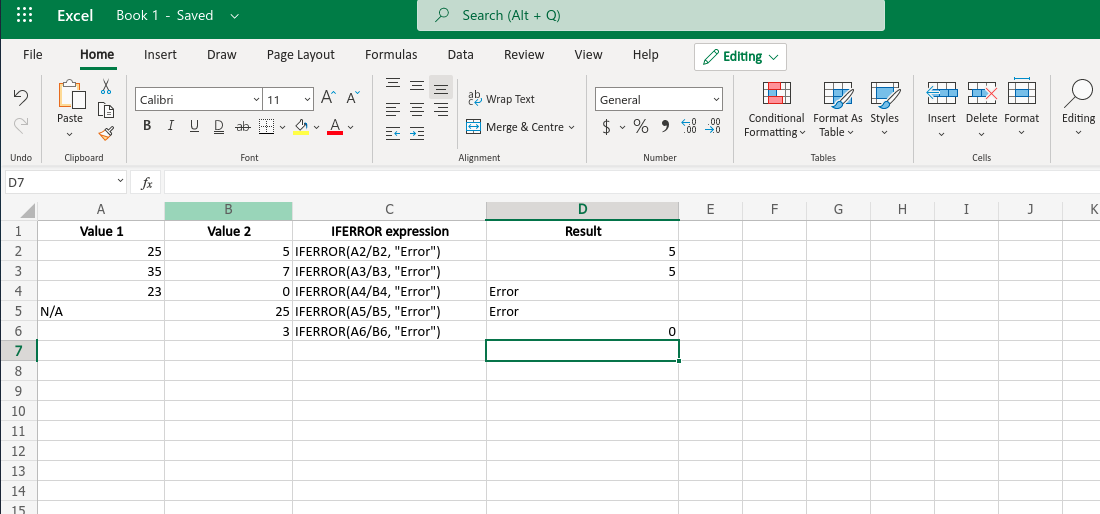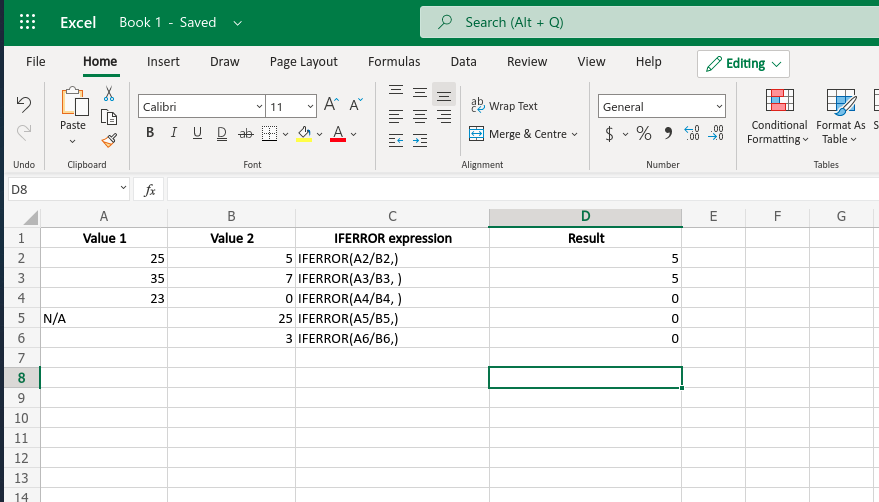# IFERROR Function in Excel With Examples

• Last Updated : 15 May, 2021

Excel is a useful tool for data handling, and it is widely used worldwide. The built-in functions make this application more user-friendly. Such a function is the IFERROR function. This function helps to trap and handles the errors while using a formula(such as dividing something by ‘0’). This function is very useful to an Excel user.

This function works exactly according to its name. It returns a custom result or a value that is specified by the user ‘IF’ an ‘ERROR’ occurs. Otherwise, it returns the result generated by the specified function or formula. This function is used to handle all types of Excel errors including #DIV/0!, #N/A, #NAME?, #NULL!, #NUM!, #REF!, and #VALUE! errors.

Syntax:

`IFERROR(value, value_if_error)`

Here, the value_if_error argument may be NULL or a Null string.

Arguments:

• value(Required): This is the value, function, or reference that will be checked by the IFERROR function for an error. This argument must be provided by the user.
• value_if_error(Required): This argument is also provided by the user. IFERROR returns this value or text or numbers if an error is found. This argument may be an empty string or null.

Return value: This function returns the contents of the value_if_error argument if an error is found. Otherwise, it returns the standard result of the specified formula or function.
Depending on the content of the value_if_error argument, this function may replace the error value with some other values, some text, logical values, or some results of another formula or function. This function returns ‘0’ if an error occurs and the value_if_error parameter is not provided. If the value is an array, this function returns an array of results containing results for each cell.

Example:

• When the value_if_error argument is provided:

Output:Output Excel screenshot

• when the value_if_error argument is not provided:

Basically in this case the function will return zero.

Output:Output Excel screenshot

My Personal Notes arrow_drop_up